# Sides of triangle

Triangle circumference with two identical sides is 117cm. The third side measures 44cm. How many cms do you measure one of the same sides?

x =  36.5 cm

### Step-by-step explanation:

Try calculation via our triangle calculator.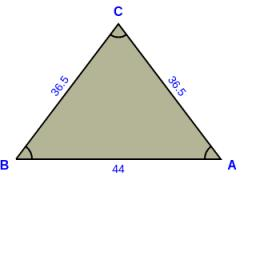Did you find an error or inaccuracy? Feel free to write us. Thank you!Tips to related online calculators

#### You need to know the following knowledge to solve this word math problem:

We encourage you to watch this tutorial video on this math problem:

## Related math problems and questions: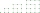The perimeter of a quadrilateral (four sided plane shape) is 125 cm . If the three known sides measure a=12 cm, b=45 cm, and c=30.5 cm, how long is the fourth side?
• TrianglesEquilateral triangle with side 40 cm has the same perimeter as an isosceles triangle with arm of 45 cm. Calculate the base x of an isosceles triangle.
• Children poolThe bottom of the children's pool is a regular hexagon with a = 60 cm side. The distance of opposing sides is 104 cm, the height of the pool is 45 cm. A) How many liters of water can fit into the pool? B) The pool is made of a double layer of plastic film
• MidpointsTriangle ABC with sides a = 5 cm, b = 3 cm, c = 40mm has a midpoint of K, L, M. How many centimeters is long perimeter of parallelogram KBLM?
• Four circles1) Calculate the circle radius if its area is 400 cm square 2) Calculate the radius of the circle whose circumference is 400 cm. 3) Calculate circle circumference if its area is 400 cm square 4) Calculate the circle's area if perimeter 400 cm.
• ParallelogramCalculate the missing side of a parallelogram, if you know the perimeter o and one side: a) o = 7.2 cm; b = 1.8 cm b) o = 5.4 cm; a = 1.9 cm
• ParallelogramThe perimeter of the parallelogram is 417 cm. The length of one side is 1.7-times longer than the length of the shorter side. What is the length of sides of a parallelogram?
• Arm-legCalculate the length of the base of an isosceles triangle with a circumference 224 cm if the arm length is 68 cm.
• The perimeter 6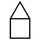The perimeter of quadrilateral is 442.95 cm. If the three known sides measure 84.56 cm,132.77 cm, and 82.75 cm, how long is the fourth side?
• The parallelogram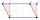The parallelogram has one side 2 dm long, which is one-sixth of its circumference. How many cm does the other side of the parallelogram measure?
• Perimeter of rhombus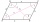The area of the rhombus is 32 cm2. One side of it measures 10 cm. The height on the other side measures 5 cm. What is the circumference of this rhombus?
• Second sideCalculate the length of the other side of the rectangle if its circumference is 60 cm and one side is 10 cm long.
• Isosceles triangleThe circumference of the isosceles triangle is 32.5 dm. Base length is 153 cm. How long is the leg of this triangle?
• Triangle ABCIn a triangle ABC side b measure 10 cm less than the side a and side b is half of the side c. Calculate the length of sides if the circumference of the triangle is 42 cm.
• Three sidesSide b is 2 cm longer than side c, side a is 9 cm shorter than side b. The triangle circumference is 40 cm. Find the length of sides a, b, c . .. .
• A cylinderA cylinder 108 cm high has a circumference of 24 cm. A string makes exactly 6 complete turns around the cylinder while its two ends touch the top and bottom. (forming a spiral around the cylinder). How long is the string in cm?
• Parallelogram perimeter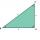The ABC triangle with sides a = 5cm, b = 3cm, c = 40mm has the center of the sides of the K,L,M. How many cm have the KBLM parallelogram perimeter?